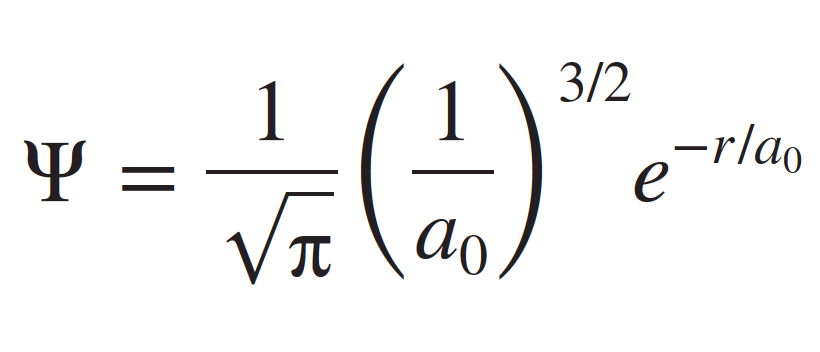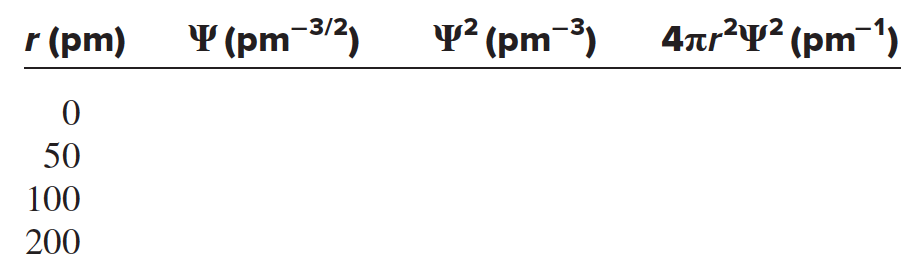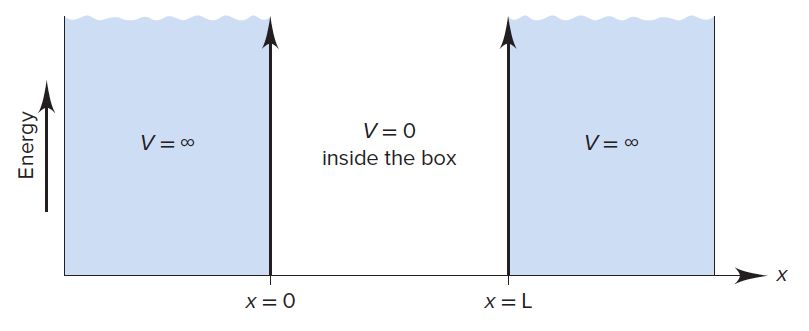# Problem: The quantum-mechanical treatment of the hydrogen atom gives this expression for the wave function, ψ, of the 1s orbital:where r is the distance from the nucleus and a0 is 52.92 pm. The probability of finding the electron in a tiny volume at distance r from the nucleus is proportional to ψ2. The total probability of finding the electron at all points at distance r from the nucleus is proportional to 4πr2ψ2. Calculate the values (to three significant figures) of ψ, ψ2, and 4πr2ψ2 to fill in the following table and sketch a plot of each set of values versus r. Compare the latter two plots with those in the figure below.

###### FREE Expert Solution
82% (228 ratings)
###### Problem Details

The quantum-mechanical treatment of the hydrogen atom gives this expression for the wave function, ψ, of the 1s orbital:where r is the distance from the nucleus and a0 is 52.92 pm. The probability of finding the electron in a tiny volume at distance r from the nucleus is proportional to ψ2. The total probability of finding the electron at all points at distance r from the nucleus is proportional to 4πr2ψ2. Calculate the values (to three significant figures) of ψ, ψ2, and 4πr2ψ2 to fill in the following table and sketch a plot of each set of values versus r. Compare the latter two plots with those in the figure below.Frequently Asked Questions

What scientific concept do you need to know in order to solve this problem?

Our tutors have indicated that to solve this problem you will need to apply the Dimensional Boxes concept. If you need more Dimensional Boxes practice, you can also practice Dimensional Boxes practice problems.

What textbook is this problem found in?

Our data indicates that this problem or a close variation was asked in Chemistry: The Molecular Nature of Matter and Change - Silberberg 8th Edition. You can also practice Chemistry: The Molecular Nature of Matter and Change - Silberberg 8th Edition practice problems.